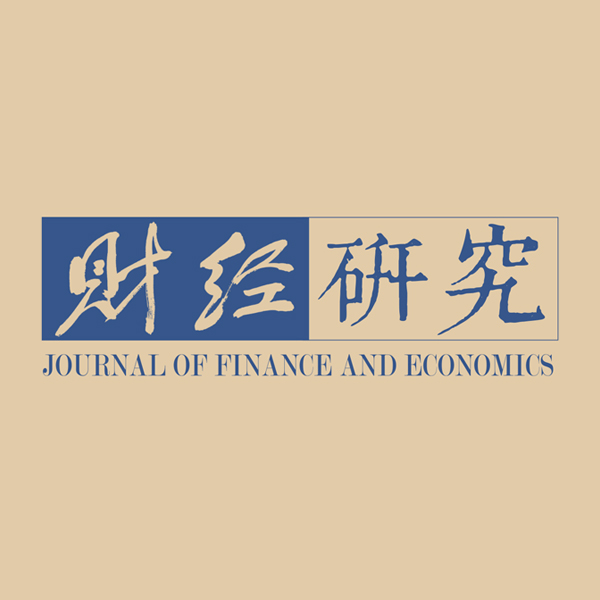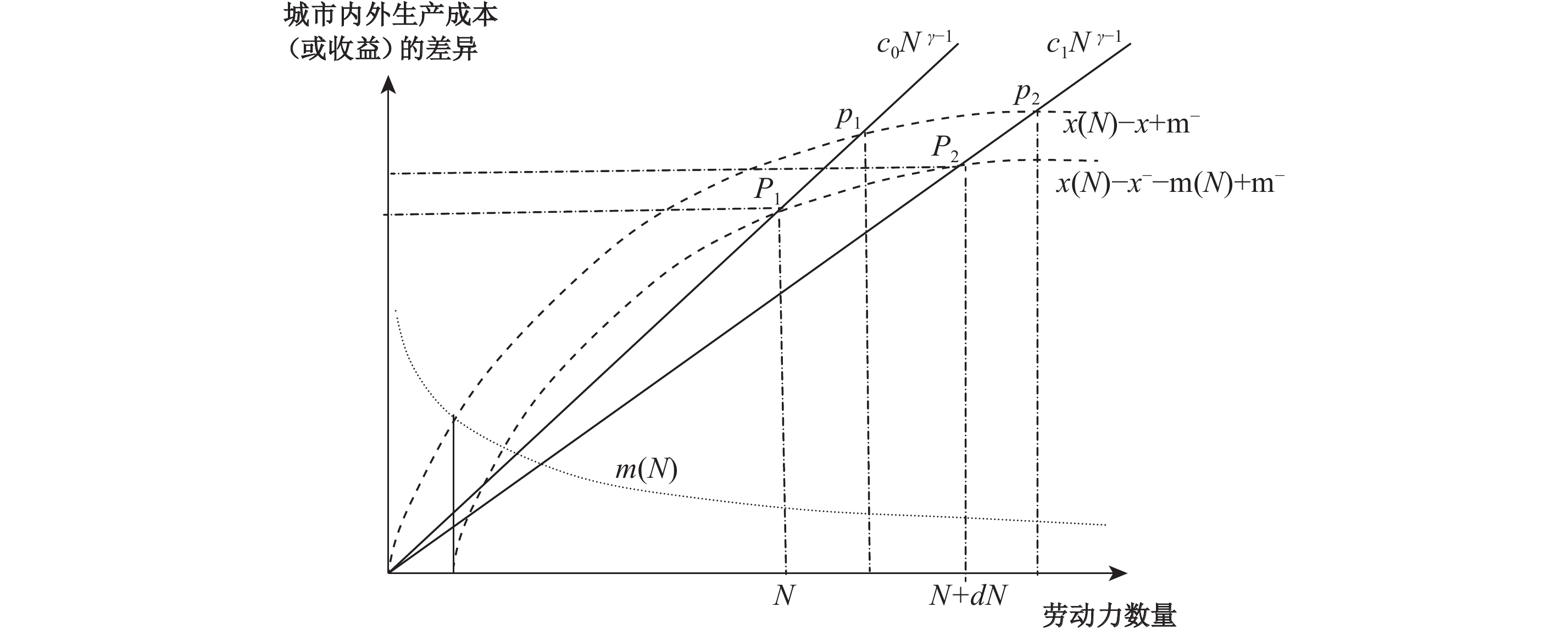﻿ 交通条件、城市规模和劳动技能匹配效应《财经研究》
2019第45卷第11期

1. 湖南大学 经济与贸易学院，长沙 410079;
2. 国家发改委宏观经济研究院 产业所，北京 100038

The Effects of Transport Infrastructure, Urban Scale and Labor Skill Matching on Firm Productivity
Zheng Tengfei1,2, Ke Shanzi11. School of Economics and Trade, Hunan University, Changsha 410079, China;
2. Academy of Macroeconomic Research, National Development and Reform Commission, Beijing 100038, China
Summary: In the past two decades, China’s labor productivity has generally increased substantially, but there are still large differences between regions and cities. The traditional neoclassical economic theory holds that the difference between factor input and agglomeration economy is the cause of the difference in labor productivity. New economic geographers emphasize that the level of transportation costs and the size of economies of scale will have different effects on the market size and labor productivity in different regions and cities. Subsequent research through formal theoretical model analysis points out that the degree of skill matching between workers and jobs affects labor productivity. Labor skill matching is a source of agglomeration economies and a cause of different location preferences of industries. This paper introduces skill matching into the model originated by Venables (2007) and uses a firm-level panel dataset to examine the complex effects of skill matching, urban transport, and city size on firm productivity in China’s cities. The study shows that transport condition, city size and skill matching have substitution effects on labor productivity; transport improvement and city expansion enhance the positive impact of skill matching on firm productivity, and the impact differs across industries and cities; transport improvement is more conducive to promoting the productivity of high-tech firms in large cities, inducing high-tech industries to agglomerate in large cities; the spatial effects of transport improvement on low-tech industries appear to be insignificant. This paper provides some empirical evidence for the development and evaluation of regional development and structural adjustment policies. In the adjustment of industrial structure, each region should take advantage of its own industry characteristics and city scale to reduce resistance, actively introduce (and move out) related industries, and promote large cities to become high-tech industrial centers, while guiding other cities to become the bases with lower production costs and higher efficiency.
Key words: skill matching    transport infrastructure    urban scale    firm productivity

$\hat z$

$c(z)$
$\rho (z)$

 $y\left( N \right) = x\left( N \right) - m\left( N \right) - c(z) - \rho (z),\;\;\;\;\;\;z \in \left[ {0,\hat z} \right]$ (1)

$\overline m$
，因此其收入为
$\overline y = \overline x - \overline m$
。空间区位均衡时，城市边缘的地租为0，城市边缘职工在城区内外就业的净收入相同，
$y = \overline y$

 $x\left( N \right) - m\left( N \right) - c(\hat z) = \overline x - \overline m$ (2)

$n\left( z \right) = k{z^\theta }$
，其中的kθ是参数，城市规模为
$N = \int_0^{\hat z} {k{z^\theta }} dz =$
${\hat z^{\left( {1 + \theta } \right)}}k/\left( {1 + \theta } \right)$
，解出城市规模为N的城区半径
$\hat z = {\left[ {N\left( {1 + \theta } \right)/k} \right]^{1/\left( {1 + \theta } \right)}}$
。令交通成本
$c\left( z \right) = c{z^\lambda }$
，参数cλ表示交通技术（如，λ=1表示交通成本是距离的线性函数）。将城市半径
$\hat z$

 $c\left( {\hat z} \right) = c{\left[ {N\left( {1 + \theta } \right)/k} \right]^{\lambda /\left( {1 + \theta } \right)}}$ (3)

$\hat z$
N的联立方程。把式（3）代入式（2），标准化k=1+θ，消去去
$\hat z$

 $x\left( N \right) - m\left( N \right) - \left( {\overline x - \overline m } \right) = c{N^{\gamma - 1}},\;\;\text{其中：}\gamma = \frac{\lambda }{{1 + \theta }} + 1$ (4)

$x\left( N \right) - \overline x = c{N^{\gamma - 1}}$
，这表明均衡时集聚经济恰好被交通成本所抵消。改善交通条件（即交通成本c下降）将导致城市均衡规模N扩大，因此，城市规模是交通条件的增函数，N(c)，
$\;({{dN} / {dc}}\;)\; < 0$

$x\left( N \right) = x\left( {N(c)} \right)$
$m\left( N \right) =$
$m\left( {N(c)} \right)$
，利用式（4）对c求导并整理后得：

 $\left[ {\left( {{x'} - {m'}} \right) - \left( {\gamma - 1} \right)c{N^{\gamma - 2}}} \right]\frac{{dN}}{{dc}} = {N^{\gamma - 1}}$ (5)图 1 劳动技能匹配

 $x\left( h \right) - \mu m\left( N \right) = x - \left( {\frac{1}{n} - \mu } \right)m\left( N \right)$ (6)

 $\mu = \frac{1}{{{\rm{2}}n}} + \frac{1}{{{\rm{2}}m\left( N \right)}}\left[ x\left( h \right){ - x} \right]$ (7)

 $l\left( h \right) = 2\bar N\mu = \frac{{\bar N}}{n} + \left[ {x\left( h \right) - x} \right]\frac{{\bar N}}{{m\left( N \right)}}$ (8)

$\bar N$

$l\left( h \right) = {{\bar N} / n}$
。标准化k=1+θ，把城市职工通勤成本加总并整理后得
${N^\gamma }c/\gamma$

$\bar N$
N，可得企业总收益：

 $Y = \frac{1}{n}\left[ {Nx\left( N \right) - Nm\left( N \right) - {(N^\gamma }c/\gamma) + \left( {\bar N - N} \right)\left( {\bar x - \bar m} \right)} \right]$ (9)

$\bar N$
$\bar x\;\text{和}\;\bar m$

$Y = F\left( {x\left( {N(c)} \right),\;m\left( {N(c)} \right),N(c),c} \right)$

 $\frac{{dY}}{{dc}} = \frac{1}{n}\left[ {N\left( {{x'} - {m'}} \right) + \left( {x(N) - m(N) - (\bar x - \bar m)} \right) - c{N^{\gamma - 1}}} \right]\frac{{dN}}{{dc}} - \frac{{{N^\gamma }}}{{n\gamma }}$ (10)

${{{N^\gamma }} / {n\gamma }}$
（即
${{d({N^\gamma }c/n\gamma )} / {dc}}$
）表示不计其他间接途径，改善交通可以直接节省市内所有职工的通勤成本。

$({{dN} / {dc}})$
dc<0而上升），且
$N(x') > 0$
，因此
$N(x')({{dN} / {dc}}) > 0$
。城市经济学的一个基本预期和以往大量经验研究都发现，由于集聚经济和集聚不经济，城市规模与人均产出率呈倒U形关系。交通条件的改善通过影响城市规模和集聚经济提高了生产率。交通基础设施对不同规模城市企业的影响存在差异，其中，交通基础设施的发展更有利于提高尚未达到最优城市规模的大城市的企业生产率。

$m' < 0$
，所以交通的改善使
$N( - m'){{(dN} / {dc}}) > 0$
。交通设施的匹配收益是城市规模和边际匹配成本共同作用的结果。城市规模越大的地区，交通基础设施改善匹配效益的潜力就越小；相反，城市规模越小，匹配成本越大，交通基础设施的匹配收益越高。

$x\left( N \right) - m\left( N \right) - \left( {\bar x - \bar m} \right) - c{N^{\gamma - 1}}$图 2 交通条件、城市规模和劳动技能匹配对生产率的影响

$\overline x - \overline m$
。设初始交通成本为起自原点的直线
${c_0}{N^{\gamma - 1}}$
c0是斜率，交通成本随城市规模而增长。交通条件改善后，斜率下降为c1，交通成本线自原点向右旋转为
${c_1}{N^{\gamma - 1}}$
。曲线
$x(N) - \overline x + \overline m$

${c_0}{N^{\gamma - 1}}$
${c_1}{N^{\gamma - 1}}$

$m(N) > 0$

$x(N) - m(N) - \bar x + \bar m$
。该曲线与两交通成本的交点（大写字母P1P2）是达到空间均衡的城市规模（NN+dN）和生产率，在此状态下，企业和职工都不再有迁移的经济激励。若交通成本不变（沿
${c_0}{N^{\gamma - 1}}$
），匹配成本m(N)使城市均衡规模N和生产率y坐标交点由小写字母p1点下降为大写的P1。由此得到理论预期，生产率是匹配成本m(N)的减函数。

（一）计量模型设定。根据以上分析，由于集聚经济和交通条件的改善均有助于劳动生产率的增长，城市规模、交通条件和技能匹配直接影响劳动生产率，但是技能错配会降低生产率。更为重要的是，劳动力在找工作时，城市规模和交通条件的改善会提高匹配程度；而且，给定城市规模和交通条件，企业可以通过培训投入来改善现有的技能匹配水平。因此，交通条件、城市规模和企业培训对企业生产率的直接影响和协同影响是本文考察的重点。同时需要说明，搜寻和培训是前后两个阶段的行为，其目的都是实现技能匹配，但是两者的作用可以替代（已达到技能匹配时）也可以叠加（尚未达到匹配时）。理论模型的构建过程力求刻画技能匹配机制，避免遗漏重要的相关变量，但均衡模型的最终结果不在于区分搜寻和培训所处的阶段，而在于揭示各变量对生产率的可能的贡献。计量模型检验的是均衡模型，所以城市规模、交通条件和企业培训投入变量的参数估计都是包含了上述两个阶段变化的最终结果。

 \begin{aligned} \ln {Y_{ijt}} = & {\beta _0} + {\beta _{\rm{1}}}\ln {C_{it}} + {\beta _2}\ln {N_{it}} + {\beta _{\rm{3}}}\ln {m_{ijt}} + {\beta _4}\ln {m_{ijt}}\ln {N_{it}} + {\beta _5}\ln {m_{ijt}}\ln {C_{it}} + {\beta _6}\ln {N_{it}}\ln {C_{it}} \\ & + {\beta _7}\ln {m_{ijt}}\ln {C_{it}}\ln {N_{it}} + {\beta _8}{\left( {\ln {N_{it}}} \right)^2} + {\beta _9}\ln {X_t} + \sum\limits_{h = 1}^H {{\lambda _h}in{d_h}} + \sum\limits_{i = 1}^n {{\lambda _i}cit{y_i}} + \sum\limits_{t = 1}^T {{\lambda _t}yea{r_t}} + {\varepsilon _{ijt}} \end{aligned} (11)

 \begin{aligned} \ln {Y_{ijt}} = & {\beta _0} + {\beta _{\rm{1}}}\ln {C_{it}} + {\beta _2}\ln {N_{it}} + {\beta _{\rm{3}}}\ln {m_{ijt}} + {\beta _4}\ln {m_{ijt}}\ln {N_{it}} + {\beta _5}\ln {m_{ijt}}\ln {C_{it}} + {\beta _6}\ln {N_{it}}\ln {C_{it}} \\ & + \sum\limits_{k = 1}^3 {\left( {{\beta _{{\rm{7k}}}}N_t^k\ln {m_{ijt}}\ln {C_{it}}} \right)} + {\beta _8}{\left( {\ln {N_{it}}} \right)^2} + {\beta _9}\ln {X_t} + \sum\limits_{h = 1}^H {{\lambda _h}in{d_h}} + \sum\limits_{i = 1}^n {{\lambda _i}cit{y_i}} + \sum\limits_{t = 1}^T {{\lambda _t}yea{r_t}} + {\varepsilon _{ijt}} \end{aligned} (12)

（二）变量和数据说明。本文数据来自国家统计局发布的《中国工业企业数据库》和《中国城市统计年鉴》。《中国工业企业数据库》中只有2004—2007年四年序列中报告了企业劳动培训支出数据，这成为本文时间序列的约束条件。工业企业数据库中有些指标存在异常值，本文样本剔除了职工人数、总资产或固定资产净值和销售额缺失的观测值。根据谢千里等（2008）的方法，剔除职工人数少于8人的观测值。本文还剔除了企业年龄大于100的个别可疑记录。由于工业企业数据库中各年收集的统计指标个数差别较大，本文选取在样本期间内持续存在的变量。稳健性检验还将使用被解释变量高低端各1%截尾后的样本。由于从2004年起，国家统计局不再收集工业增加值和工业总产值数据，本文参照聂辉华等（2012）的方法，根据会计准则估算，工业增加值=产品销售额−期初存货+期末存货−工业中间投入+增值税。劳动生产率用企业增加值与企业职工数的比值表示。

 变量 均值 标准差 极小值 极大值 Prod（企业劳动生产率，元/人） 95 166 104 177 2 301 697 923 TFP（全要素生产率对数值） 3.2842 1.0277 −3.5339 4.6493 Street（路网密度，km/km2） 8.04 3.62 1.07 22.40 Match（培训投入，千元/人） 0.132 0.62 0 61.910 Pop（城市规模，万人） 270.37 315.96 14.08 1 526.02 Size（企业规模，人） 243.48 936.10 8 108 525 Age（企业年龄，年） 8.64 8.79 1 99 St（每万人大学生数，人/万人） 471 333.96 1 2 284 Kn（科技支出占财政支出比重） 0.0149 0.0452 1.32e-06 0.328 4

（一）交通基础设施、城市规模和培训投入对中国企业生产率影响的计量检验。除了虚拟变量，文中变量均采用对数形式。所有模型的Hausman检验都拒绝了随机效应原假设，表明未控制的企业特征与某些解释变量相关，必须控制这些企业的固定效应（FE）才能得到一致性参数估计。此外，一些地区推行的特定产业政策影响相关产业的企业生产率， 所以回归模型引入了省份与产业固定效应的交互项（29×29个虚拟变量，其中部分变量因共线性而被自动删除）以控制地区层面的特定产业政策差异。为了检验交通条件、城市规模和技能匹配对企业生产率的影响，我们逐步引入城市路网密度、城市规模和技能匹配（用企业培训投入测度）的交互项，检验三个不同的固定效应模型。对中国诸多城市的大量企业来说，城市规模和培训投入两变量对企业生产率有独立的作用。同时，城市规模和培训投入这两个变量的变化相互作用，很可能影响各自对企业生产率的作用。如果城市人口增长显著增强了企业培训投入对生产率的作用（即交互项的计量结果是显著的正参数），根据经济学一般逻辑，城市人口规模是企业培训投入的互补因素。相反，如果城市人口增长削弱了企业培训投入对生产率的作用（交互项有显著的负参数），根据经济学逻辑，城市人口规模和企业培训投入就很可能是替代因素。我们进而推测其机制，合乎逻辑和常理的解释是，具有城市人口规模优势的企业不需要大量内部培训即可获得较好的技能匹配，而城市人口规模越小的企业越需要依靠内部培训才可获得所需的技能匹配。我们根据同样逻辑解释交通条件和技能培训交互项的实证结果。同时，为了控制城市规模可能带来的内生性问题，所有回归方程中使用城市规模滞后一期的数据。表2报告了方程（11）的FE模型估计结果。

 1.lnprod 2.lnprod 3.lnprod 4.lnprod lnStreet 0.0776***（0.0083） 0.0647***（0.0088） −0.2812***（0.0315） −0.2422***（0.0326） lnMatch 0.0029***（0.0005） 0.0207***（0.0032） 0.0294***（0.0032） 0.0374***（0.0037） lnStreet×lnMatch −0.0041***（0.0008） −0.0074***（0.0008） −0.0096***（0.0010） lnPop×lnMatch −0.0014***（0.0005） −0.0014***（0.0005） −0.0040***（0.0008） lnStreet×lnPop 0.0653***（0.0059） 0.0629***（0.0060） lnPop×lnStreet×lnMatch 0.0010***（0.0002） lnPop 1.6870***（0.0811） 1.5544***（0.1246） 1.5185***（0.0818） 1.5041***（0.0819） （lnPop）2 −0.1493***（0.0074） −0.1446***（0.0109） −0.1333***（0.0075） −0.1319***（0.0075） lnSize 0.2356***（0.0036） 0.1850***（0.0036） 0.2310***（0.0036） 0.2307***（0.0036） lnAge 0.1962***（0.0045） 0.0497***（0.0051） 0.1841***（0.0045） 0.1832***（0.0045） lnSt 0.1991***（0.0061） 0.0080（0.0078） 0.2253***（0.0065） 0.2246***（0.0065） lnKn 0.0482***（0.0015） 0.0449***（0.0031） 0.0480***（0.0015） 0.0483***（0.0015） Constant −4.2243***（0.2242） −2.3870***（0.3535） −3.8506***（0.2254） −3.8592***（0.2254） 地区产业差异 控制 控制 控制 控制 Observations 319 925 319 925 319 925 319 925 R-squared 0.1154 0.1155 0.1158 0.1159 注：括号内为标准误，***、**和*分别表示p<0.01、p<0.05 和p<0.1。下表同。

（二）交通条件、城市规模和培训投入对不同技术行业和不同规模城市企业生产率的影响。不同产业具有各自的技术特点。本节根据《高技术产业统计分类目录》和OECD产业分类标准划分高、中、低技术三类产业，附表1列出了每类包含的两位码行业。高技术产业技术含量高，技术更新快，产品附加值高，需要专业知识型劳动者，同时必须支付较高的薪酬；相反，低技术产业倾向于采用成熟和稳定的技术，雇工进行更多的常规生产，职工的薪酬也相对较低；中等技术产业的技术含量则介于高、低技术产业之间。各类产业因技术特征和对要素投入的要求不同而有不同的区位偏好。大城市具有较大的专业劳动力市场和产品市场、较完整的上下游相关产业和较迅速的技术信息传播，因而成为高技术产业的首选之地，而中小城市则因土地和一般劳力等要素价格较低而成为低技术产业的集聚地。交通条件的改善不仅有利于产品运输和信息传播，也有利于劳动力流动。表3报告了方程（12）的分产业固定效应回归结果。

 高技术 中技术 低技术 lnStreet −0.6262***（0.2177） −0.0732（0.0764） −0.1718*（0.0934） lnMatch 0.0298（0.0264） 0.0507***（0.0079） 0.0445***（0.0095） lnStreet×lnMatch −0.0054（0.0035） −0.0092***（0.0011） −0.0059***（0.0013） lnPop×lnMatch −0.0145***（0.0047） −0.0049***（0.0015） −0.0049***（0.0018） lnStreet×lnPop 0.1154***（0.0399） 0.0213（0.0145） 0.0494***（0.0181） N1×lnStreet×lnMatch −0.0079** （0.0033） −0.0027***（0.0009） 0.0013 （0.0010） N3×lnStreet×lnMatch 0.0079** （0.0035） 0.0036***（0.0011） 0.0016 （0.0014） lnPop 2.6191***（0.5507） 1.9522***（0.1782） 2.3910*** （0.1975） （lnPop）2 −0.2309***（0.0474） −0.1581***（0.0154） −0.2057*** （0.0173） lnSize 0.2272***（0.0157） 0.2535*** （0.0049） 0.1988***（0.0057） lnAge 0.1257***（0.0187） 0.1576***（0.0062） 0.2294*** （0.0074） lnSt 0.2243***（0.0278） 0.2364***（0.0091） 0.2122*** （0.0104） lnKn 0.0304***（0.0056） 0.0520***（0.0020） 0.0446*** （0.0024） Constant −6.8244***（1.5889） −5.5849***（0.5126） −6.1113*** （0.5609） 地区产业差异 控制 控制 控制 Observations 22 825 174 415 122 685 R-squared 0.1205 0.1032 0.1010 MEM1 0.0031 0.0378 0.0812 MEM2 0.0042 0.0381 0.0811 MEM3 0.0052 0.0385 0.0812
1 高中低技术产业分类
 分类 产业（SIC代码） 高技术产业 核燃料加工（2530）、信息化学品制造（2665）、医药制造业（27）、医疗仪器设备及器械制造（368）、航空航天器制造（376）、通信设备、计算机及其他电子设备制造业（40）、通用仪器仪表制造（411）、专用仪器仪表制造（412）、光学仪器制造（4141）、复印和胶印设备制造（4154）、计算器及货币专用设备制造（4155）、其他仪器仪表的制造及修理（4190） 中技术产业 文教体育用品制造业（24）、化学原料及化学制品制造业（26，不含信息化学品制造2665）、化学纤维制造业（28）、塑料制品业（30）、非金属矿物制品业（31）、有色金属冶炼及压延加工业（33）、金属制品业（34）、通用设备制造业（35）、专用设备制造业（36，除医疗仪器设备及器械制造368）、交通运输设备制造业（37，除航空航天器制造376）、电气机械及器材制造业（39）、眼镜制造（4142）、文化、办公用机械制造（415，除复印和胶印设备制造4154、计算器及货币专用设备制造4155）、工艺品及其他制造业（42） 低技术产业 农副食品加工业（13）、食品制造业（14）、饮料制造业（15）、烟草制品业（16）、纺织业（17）、纺织服装鞋帽制造业（18）、皮革、毛皮、羽毛（绒）及其制品业（19）、木材加工及木竹藤棕草制品业（20）、家具制造业（21）、造纸及纸制品业（22）、印刷业和记录媒介的复制（23）、石油加工、炼焦及核燃料加工业（25，不含核燃料加工2530）、橡胶制品业（29）、黑色金属冶炼及压延加工业（32）、废弃资源和废旧材料回收加工业（43）

$\ln Match = \overline {\ln Match}$
）交通条件对中小城市、大城市和特大城市企业生产率的影响。以高技术行业为例，该行业的劳动培训投入均值为0.1299，所以交通基础设施对中小城市高技术产业生产率的影响为MEM1=−0.6262−0.0054×0.1299+0.1154 ×5.4686−0.0079×0.1299=0.0031。同理，我们可以计算MEM2和MEM3。比较高、中、低三个产业模型中的MEM1、MEM2和MEM3值，发现改善交通条件对三类技术行业的企业生产率都有促进作用，但在中技术行业未通过显著性检验。其中：低技术行业受益更明显，因为与高技术职工相比，中低技术行业职工收入较低，所以其通勤距离受交通成本的影响更大。高技术模型中MEM1和MEM3的值表明，在特大城市地区改善交通条件更加有利于高技术产业改善技能匹配、提高生产率。据此可以判断，高技术产业从中小城市向特大城市集聚可以利用更便利的交通和更大的劳动市场获得更大的效益。需要指出的是，交通条件和培训投入对中小城市中低技术产业的生产率与大城市和特大城市并无显著差别。日常观察和城市经济学原理都指出特大城市除了集聚经济外，也伴生有集聚非经济，尤其是持续上涨的土地和人力要素成本、生活成本以及拥堵效应等都不利于传统的技术成熟的劳动密集型产业经营和发展。因而，低技术产业应当从特大城市转移到土地和劳动要素价格较低的其他城市。一些研究（Duranton和Puga，2005；赵勇和白永秀，2012）已经发现，交通通讯的发展导致了企业总部和生产部门的分离，使中心城市主要承担了管理和研发的功能，而外围城市则承担了加工和制造的功能。本研究从产业技术特征切入，发现了类似的统计规律。

（三）稳健性检验。为了检验上述结论的稳健性，我们用厂商全要素生产率TFP替换劳动生产率重估上述模型。虽然劳动生产率的差别是TFP差别的重要决定因素，但是其他要素（例如资本）的效益差别也影响着TFP，所以我们主要关注两组方程参数估计值的正负和显著性，而非每个估计值的大小。TFP可以用不同的方法测度。鲁晓东和连玉君（2012）分析和比较了TFP的最小二乘估计、固定效应估计、OP估计和LP估计，发现最小二乘法和固定效应估计法都不能解决同时性偏差问题，而OP估计无法处理投资额为0的样本。因而，本文使用LP法估计企业的全要素生产率。实证结果可能受一些极端值的影响，为检验上述方程的稳健性，我们还使用被解释变量lntfp高低端各1%截尾样本重估方程。表4报告了交通条件和企业培训对全国所有制造业和三类技术产业企业生产率影响的估计结果。

 全国样本 高技术行业 中技术行业 低技术行业 lnStreet −0.1999***（0.0524） −0.6708***（0.2090） −0.1833**（0.0724） −0.2437***（0.0883） lnMatch 0.0306***（0.0035） 0.0204（0.0253） 0.0402***（0.0075） 0.0338***（0.0090） lnStreet×lnMatch −0.0095***（0.0009） −0.0054（0.0033） −0.0090***（0.0011） −0.0058***（0.0013） lnPop×lnMatch −0.0033***（0.0007） −0.0102**（0.0045） −0.0029**（0.0014） −0.0029*（0.0017） lnStreet×lnPop 0.0505***（0.0100） 0.1267***（0.0383） 0.0408***（0.0137） 0.0594***（0.0171） lnPop×lnStreet×lnMatch 0.0009***（0.0002） N1×lnStreet×lnMatch −0.0036（0.0034） −0.0022**（0.0009） 0.0004（0.0010） N3×lnStreet×lnMatch 0.0063**（0.0031） 0.0026**（0.0011） 0.0010（0.0014） lnPop 2.2784***（0.1200） 2.1709***（0.5276） 2.0482***（0.1688） 2.5843***（0.1867） （lnPop）2 −0.1923***（0.0104） −0.1922***（0.0454） −0.1679***（0.0146） −0.2233***（0.0164） lnSize 0.3133***（0.0034） 0.3623***（0.0150） 0.3377***（0.0046） 0.2723***（0.0053） lnAge 0.2078***（0.0043） 0.1715***（0.0179） 0.1818***（0.0059） 0.2547***（0.0070） lnSt 0.2417***（0.0063） 0.2416***（0.0266） 0.2574***（0.0086） 0.2178***（0.0098） lnKn 0.0397***（0.0014） 0.0240***（0.0054） 0.0451***（0.0019） 0.0337***（0.0023） Constant −4.5072***（0.3443） −4.4005***（1.5226） −4.2274***（0.4858） −4.7296***（0.5303） 地区产业差异 控制 控制 控制 控制 Observations 313 526 22 368 170 927 120 231 R-squared 0.1541 0.1128 0.1624 0.1493

（四）企业培训内生性检验。企业培训支出有利于提高生产率，而企业培训支出也可能取决于生产率水平，企业培训可能具有内生性。现实中，生产率高的企业有更多的财力进行企业职工培训，而生产率低的企业则在提高职工技能上有更强烈的紧迫感。因此，两类企业在技能培训方面的内生性有正负抵消的趋势。无论内生性是正或是负，因为企业今年的生产率很难影响去年的培训支出，使用滞后一期的技能培训能进一步削弱内生影响。表5报告了估计结果，其中滞后一期变量加前缀“L.”标识。

 全国样本 高技术行业 中技术行业 低技术行业 lnStreet −0.1835***（0.0347） −0.1381（0.1321） −0.2287***（0.0471） −0.1162**（0.0582） L.lnMatch 0.6002***（0.2144） 1.0832（0.7378） 0.4133（0.2825） 0.8070**（0.4012） lnStreet×l. lnMatch −0.2596**（0.1039） −0.4622（0.3555） −0.2072（0.1349） −0.3663*（0.1980） lnPop×l.lnMatch −0.1119***（0.0396） −0.2067*（0.1253） −0.0551（0.0521） −0.1871**（0.0771） lnStreet×lnPop 0.0441***（0.0066） 0.0273（0.0242） 0.0546***（0.0089） 0.0305***（0.0113） lnPop×lnStreet×l.lnMatch 0.0046**（0.0019） N1×lnStreet×l.lnMatch −0.0103（0.0117） −0.0080***（0.0028） −0.0013（0.0075） N3×lnStreet×l.lnMatch 0.0098**（0.0046） 0.0060**（0.0024） 0.0020（0.0030） lnPop 1.3976***（0.1223） 1.4406***（0.1759） 1.3378**（0.5559） 1.4099***（0.1829） （lnPop）2 −0.1141***（0.0107） −0.1149***（0.0153） −0.1177**（0.0480） −0.1164***（0.0162） lnSize 0.1795***（0.0042） 0.2044***（0.0179） 0.1999***（0.0058） 0.1489***（0.0066） lnAge 0.2179***（0.0062） 0.1928***（0.0251） 0.1936***（0.0084） 0.2577***（0.0099） lnSt 0.0891***（0.0079） 0.1161***（0.0346） 0.0869***（0.0110） 0.0810***（0.0121） lnKn 0.0560***（0.0014） 0.0348***（0.0053） 0.0622***（0.0019） 0.0507***（0.0023） Constant −2.4333***（0.3413） −2.5267（1.5654） −2.7544***（0.4932） −2.1705***（0.5054） 地区产业差异 控制 控制 控制 控制 Observations 239 423 17 112 130 205 92 106 R-squared 0.1121 0.1084 0.1087 0.1096

① 数据来源于《中国城市统计年鉴》和《2016智能出行大数据报告》。

② 中国企业报道：http://www.ceccen.com/toutia oxinwen/1458701214.html；搜狐网、商务部网站。

${\int\limits_0^{\hat z} {k{z^\theta }c{z^\lambda }dz} = \dfrac{{ck{{\hat z}^{\left( {1 + \theta + \lambda } \right)}}}}{{1 + \theta + \lambda }} = \dfrac{{ck}}{{1 + \theta + \lambda }}{\left[ {\dfrac{{N\left( {1 + \theta } \right)}}{k}} \right]^{\left( {1 + \theta + \lambda } \right)/\left( {1 + \theta } \right)}} = {N^\gamma }c/\gamma }$

④ 高新技术产业需要大量高技能人才和便利的交通和信息交往，因此往往集聚在大城市。技术成熟的传统产业需要成本较低的土地、原材料和一般劳动力，因而偏好在要素成本较低的二、三线城市。

⑤ 例如，国务院关于印发“十三五”国家战略性新兴产业发展规划的通知指出，山东半岛城市群重点发展生物医药、高端装备制造、新一代信息技术、新材料等产业，而福建重点发展海峡西岸地区生物、海洋、集成电路等产业，以及东北地区大力发展机器人及智能装备、光电子、生物医药及医疗器械等产业。

⑥ 令含城市人口规模的各项lnPop、lnPop×lnMatch、lnStreet×lnPop、lnPop×lnStreet× lnMatch和lnPop二次项的系数分别为a1a2a3a4b，则城市最佳规模的一阶条件为（a1+a2lnMatch+a3lnStreet+a4lnMatch×lnStreet）/（-2b）。由于回归结果中，a3a4的参数估计均显著为正、b显著为负，故lnStreet值越大，城市最优规模也越大。

⑦ 高技术行业中交通条件和技能培训交互项（lnStreet× lnMatch）的t=1.55（即P=0.121，否定零假设的概率87.9%），所以参数估计有一定意义。

  柯善咨, 赵曜. 产业结构、城市规模与中国城市生产率[J]. 经济研究, 2014(4): 76–88，115. DOI:10.3969/j.issn.1673-291X.2014.04.034  柯善咨, 郑腾飞. 中国城市车辆密度、劳动生产率与拥堵成本研究[J]. 中国软科学, 2015(3): 65–79. DOI:10.3969/j.issn.1002-9753.2015.03.007  林理升, 王晔倩. 运输成本、劳动力流动与制造业区域分布[J]. 经济研究, 2006(3): 115–125. DOI:10.3969/j.issn.1005-913X.2006.03.047  鲁晓东, 连玉君. 中国工业企业全要素生产率估计: 1999—2007[J]. 经济学（季刊）, 2012(2): 541–558.  聂辉华, 江艇, 杨汝岱. 中国工业企业数据库的使用现状和潜在问题[J]. 世界经济, 2012(5): 142–158.  孙晓华, 王昀. 企业规模对生产率及其差异的影响——来自工业企业微观数据的实证研究[J]. 中国工业经济, 2014(5): 57–69.  谢千里, 罗斯基, 张轶凡. 中国工业生产率的增长与收敛[J]. 经济学（季刊）, 2008(3): 809–826.  张光南, 宋冉. 中国交通对" 中国制造”的要素投入影响研究[J]. 经济研究, 2013(7): 63–75. DOI:10.3969/j.issn.1002-5863.2013.07.030  赵勇, 白永秀. 中国城市群功能分工测度与分析[J]. 中国工业经济, 2012(11): 18–30.  钟昌标, 黄远浙, 刘伟. 外资进入速度、企业异质性和企业生产率[J]. 世界经济, 2015(7): 53–72.  周黎安, 张维迎, 顾全林, 等. 企业生产率的代际效应和年龄效应[J]. 经济学（季刊）, 2007(4): 1297–1318.  Brandt L, Luhang W, Yifan Z. Productivity in Chinese industry: 1998-2013[J]. Working Paper, 2017.  Duranton G, Puga D. Micro-foundations of urban agglomeration economies[J]. Handbook of Regional and Urban Economics, 2004, 4: 2063–2117. DOI:10.1016/S1574-0080(04)80005-1  Duranton G, Puga D. From sectoral to functional urban specialisation[J]. Journal of Urban Economics, 2005, 57(2): 343–370. DOI:10.1016/j.jue.2004.12.002  Gautier P A, Zenou Y. Car ownership and the labor market of ethnic minorities[J]. Journal of Urban Economics, 2010, 67(3): 392–403. DOI:10.1016/j.jue.2009.11.005  Gollin D, Rogerson R. Productivity, transport costs and subsistence agriculture[J]. Journal of Development Economics, 2014, 107: 38–48. DOI:10.1016/j.jdeveco.2013.10.007  Graham D J. Variable returns to agglomeration and the effect of road traffic congestion[J]. Journal of Urban Economics, 2007, 62(1): 103–120. DOI:10.1016/j.jue.2006.10.001  Graham D J, Gibbons S, Martin R. Transport investment and the distance decay of agglomeration benefits[R]. Centre for Transport Studies, 2009.  Helsley R W, Strange W C. Matching and agglomeration economies in a system of cities[J]. Regional Science and Urban Economics, 1990, 20(2): 189–212. DOI:10.1016/0166-0462(90)90004-M  Marshall A. Principles of economics: An introductory volume[M]. London: Macmillan, 1890.  Phillips D C. Getting to work: Experimental evidence on job search and transportation costs[J]. Labour Economics, 2014, 29: 72–82. DOI:10.1016/j.labeco.2014.07.005  Tikoudis I, Sundberg M, Karlström A. The effects of transport infrastructure on regional economic development: A simu- lated spatial overlapping generations model with heterogenous skill[J]. Journal of Transport and Land Use, 2012, 5(2): 77–101.  Torfs W, Zhao L Q. Everybody needs good neighbors? Labor mobility costs, cities and matching[J]. Regional Science and Urban Economics, 2015, 55: 39–54. DOI:10.1016/j.regsciurbeco.2015.08.005  Venables A J. Evaluating urban transport improvements: Cost-benefit analysis in the presence of agglomeration and income taxation[J]. Journal of Transport Economics and Policy, 2007, 41(2): 173–188.